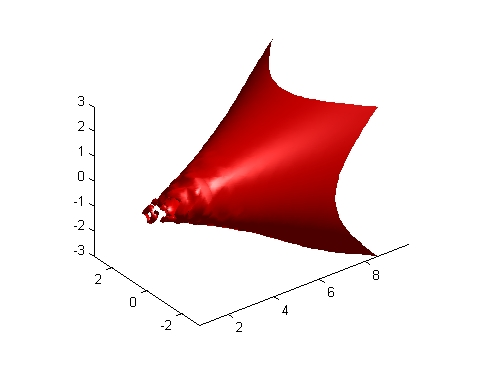MATLAB Function Referenceisosurface

Extract isosurface data from volume data

Syntax

• ```fv = isosurface(X,Y,Z,V,isovalue)
fv = isosurface(V,isovalue)
fv = isosurface(X,Y,Z,V), fv = isosurface(X,Y,Z,V)
fvc = isosurface(...,colors)
fv = isosurface(...,'noshare')
fv = isosurface(...,'verbose')
[f,v] = isosurface(...)
isosurface(...)
```

Description

```fv = isosurface(X,Y,Z,V,isovalue) ``` computes isosurface data from the volume data `V` at the isosurface value specified in `isovalue`. That is, the isosurface connects points that have the specified value much the way contour lines connect points of equal elevation.

The arrays `X`, `Y`, and `Z` define the coordinates for the volume `V`. The structure `fv` contains the faces and vertices of the isosurface, which you can pass directly to the `patch` command.

```fv = isosurface(V,isovalue) ``` assumes the arrays `X`, `Y`, and `Z` are defined as `[X,Y,Z] = meshgrid(1:n,1:m,1:p)` where `[m,n,p] = size(V)`.

```fvc = isosurface(...,colors) ``` interpolates the array `colors` onto the scalar field and returns the interpolated values in the `facevertexcdata` field of the `fvc` structure. The size of the `colors` array must be the same as `V`. The `colors` argument enables you to control the color mapping of the isosurface with data different from that used to calculate the isosurface (e.g., temperature data superimposed on a wind current isosurface).

```fv = isosurface(...,'noshare') ``` does not create shared vertices. This is faster, but produces a larger set of vertices.

```fv = isosurface(...,'verbose') ``` prints progress messages to the command window as the computation progresses.

```[f,v] = isosurface(...) ``` returns the faces and vertices in two arrays instead of a struct.

```isosurface(...) ``` with no output arguments creates a patch using the computed faces and vertices.

Special Case Behavior -- isosurface Called with No Output Arguments

If there is no current axes and you call `isosurface` with without assigning output arguments, MATLAB creates a new axes, sets it to a 3-D view, and adds lighting to the isosurface graph.

Remarks

You can pass the `fv` structure created by `isosurface` directly to the `patch` command, but you cannot pass the individual faces and vertices arrays (`f`, `v`) to `patch` without specifying property names. For example,

• ```patch(`isosurface(X,Y,Z,V,isovalue)`)
```

or

• ```[f,v] = isosurface(`X,Y,Z,V,isovalue)`;
patch('Faces',f,'Vertices',v)
```

Examples

This example uses the flow data set, which represents the speed profile of a submerged jet within an infinite tank (type `help` `flow` for more information). The isosurface is drawn at the data value of -3. The statements that follow the `patch` command prepare the isosurface for lighting by

• Recalculating the isosurface normals based on the volume data (`isonormals`)
• Setting the face and edge color (`set`, `FaceColor`, `EdgeColor`)
• Specifying the view (`daspect`, `view`)
• Adding lights (`camlight`, `lighting`)
• ```[x,y,z,v] = flow;
p = patch(isosurface(x,y,z,v,-3));
isonormals(x,y,z,v,p)
set(p,'FaceColor','red','EdgeColor','none');
daspect([1 1 1])
view(3); axis tight
camlight
lighting gouraud```

See Also

`isonormals`, `shrinkfaces`, `smooth3`, `subvolume`

Connecting Equal Values with Isosurfaces for more examples

Volume Visualization for related functions

© 1994-2005 The MathWorks, Inc.